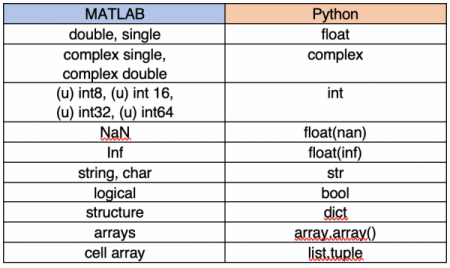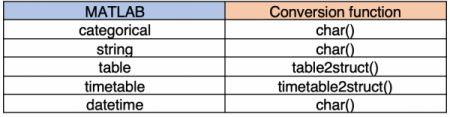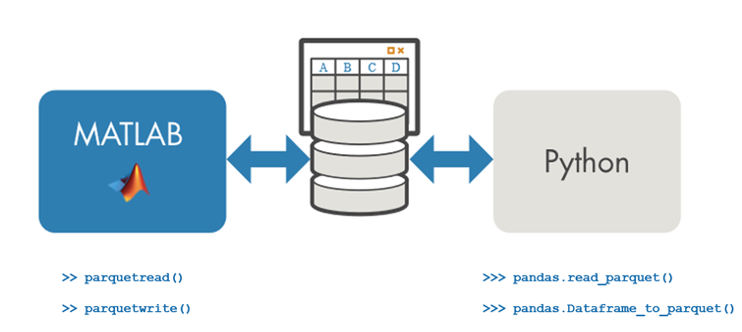# Using MATLAB and Python Together

Today’s blog is written by Heather Gorr, Product Marketing for MATLAB, and Deepak Bhatia, Education Marketing at MathWorks. In this blog, they share some important tips that will help you use MATLAB & Python together.

You’ve heard it before, MATLAB vs. Python (vs. R vs. <insert any other language>) but we’re going to talk about using MATLAB and Python together! (It can happen! And it does!) If you are a student, work in academia, or industry, you have probably encountered situations where you need to integrate work from more than one language. This is common in engineering and scientific applications, especially when they involve multiple teams and hardware needs. Such collaboration helped researchers at MIT CSAIL leverage strengths of MATLAB and C++ together to detect color and movement changes imperceptible to the naked eye.

There’s a large community working on cool algorithms, teaching, and sharing examples in both languages – so why not take advantage of all this excellent work, independent of language preference!? For example, MATLAB and Python were used in building this air quality prediction app and this sentiment analysis algorithm. The two languages are often used together for AI applications (so frequently that there are direct importers and exporters for deep learning networks through MATLAB, ONNX and TensorFlow).

This blog will show you how to use MATLAB and Python together (in peace and harmony). We’ll assume a beginner-level background in both languages and provide links to more advanced topics.

# The Basics

First, let’s get the requirements out of the way. We’ll need a recent version of Python and MATLAB R2014b or later (sounds like a good time to upgrade to R2020a!). Check here for version specifics.

This might sound obvious, but we’ll also make sure our code is accessible by both MATLAB and Python. The path can be updated easily from both languages.

# Calling Python from MATLAB

Before we dive in, let’s confirm that MATLAB can find the Python interpreter. We can do this in MATLAB with the pyenv function:

>> pyenv
ans =
PythonEnvironment with properties:

Version: "3.6"
Executable: "C:\Python36\WPy-3670\python-3.6.7.amd64\python.EXE"
Library: "C:\Python36\WPy-3670\python-3.6.7.amd64\python36.dll"
Home: "C:\Python36\WPy-3670\python-3.6.7.amd64"
ExecutionMode: OutOfProcess
ProcessID: "20980"
ProcessName: "MATLABPyHost"

This returns the Python version and environment settings, which can also be modified through the pyenv function.

Now that we can access Python, let’s use it! We’ll try the sqrt function from the math library to get the hang of it. In Python, we call it like this:

>>> import math
>>> math.sqrt(42)
6.48074069840786

To call the same Python function from MATLAB, we can use the following:

>> py.math.sqrt(42)
ans =
6.480740698407860

We used format long to display the same precision in MATLAB and Python.

Now, let’s generalize the behavior a bit. Python modules and functions are accessed using the following syntax:

>> py.module_name.function_name

User-defined modules are called in the same way. For example, the weather.py module in the air quality app includes functions which read weather data for a given location through a web API:

>> data = py.weather.get_current_weather("Boston","US",key)
data =
Python dict with no properties.

{'coord': {'lon': -71.06, 'lat': 42.36}, 'weather': [{'id': 804, 'main': 'Clouds', 'description': 'overcast clouds', 'icon': '04n'}], 'base': 'stations', 'main': {'temp': 53.2, 'feels_like': 34.9, 'temp_min': 51.01, 'temp_max': 55, 'pressure': 1003, 'humidity': 46}, 'visibility': 16093, 'wind': {'speed': 26.4, 'deg': 230, 'gust': 34.45}, 'clouds': {'all': 90}, 'dt': 1587342601, 'sys': {'type': 1, 'id': 3486, 'country': 'US', 'sunrise': 1587290159, 'sunset': 1587339006}, 'timezone': -14400, 'id': 4930956, 'name': 'Boston', 'cod': 200}

Notice that the output is a Python dictionary (in MATLAB!) We could convert this to a MATLAB type (more on data types to come), but for now we will continue to work with it directly. Let’s pull out the information of interest using another function from the module:

>> weatherData = py.weather.parse_current_json(data)
data =
Python dict with no properties.

{'temp': 39.31, 'feels_like': 31.44, 'temp_min': 37, 'temp_max': 41, 'pressure': 1010, 'humidity': 80, 'speed': 8.05, 'deg': 340, 'city': 'Boston', 'lat': 42.36, 'lon': -71.06, 'current_time': '2020-04-18 20:48:00.985146'}

We can also index into the dictionary to recall specific values:

>> T = weatherData{"temp"}
T = 39.3100

Notice that we used the curly brackets {} instead of parentheses (). In MATLAB, curly brackets are often used when accessing values from heterogeneous data types like cell arrays and tables. An easy way to remember is when you use (), you get a subset of a larger data set of the same type i.e. cell array or table. Conversely, if you use {}, you get the indexed value in its original data type i.e. double, string, char etc. Therefore, in the above example, a dict is a heterogenous data type and we will use {} to get the temperature value as a double. This example shows indexing into a Python dict.

Now that we’re getting the idea of syntax, let’s talk about another difference in calling functions. Say we wanted to change the units of the weather data. In Python, the get_forecast function accepts standard Python keyword arguments, as shown in the last argument here:

>>> forecast = weather.get_forecast("Boston","US",key,units="metric")

In MATLAB, these are passed as name-value pairs with the pyargs function:

>> forecast = py.weather.get_forecast("Boston","US",key,pyargs("units","metric")

Now that we understand how to adapt the Python syntax and call functions from MATLAB. Let’s try it the other way around! 😊

# Calling MATLAB from Python

The MATLAB Engine API for Python enables calling MATLAB as a computation engine so we can use our favorite MATLAB functions from Python. First, we need to install it via the Python package included with MATLAB. Execute the following commands in an OS prompt:

$cd "matlabroot/extern/engines/python"$ python setup.py install

matlabroot” is the directory where MATLAB is installed (check by calling >> matlabroot in MATLAB). Check this doc page for more info on setup.

Let’s get down to the fun part! To call MATLAB functions from Python, first import and start the engine (we could also use a current session of MATLAB if there is already one already running):

>>> import matlab.engine
>>> eng = matlab.engine.start_matlab()

Now that the engine is running, let’s call the square root function:

>>> x = eng.sqrt(42.0)
6.48074069840786

Note that we are calling the sqrt function with 42.0 and not simply 42. Stay tuned to learn why this is important in the next section.

MATLAB functions are called with their native syntax, but there are some distinct differences. One difference is in the way multiple outputs are captured. For example, in the sentiment analysis algorithm, the MATLAB code returns multiple outputs for the sentiment and the scores. We need to specify the number of outputs using nargout:

>>> [sentiment,scores] = eng.sentimentAnalysis(text,nargout=2)
Positive
[[0.0,0.510948896408081,0.48905110359191895]]

Similarly, if the MATLAB function returns no outputs (say the function writes results to a file), you need to pass nargout=0.

When we’re finished, we should stop the MATLAB engine to free up system resources:

>>> eng.exit()

To call MATLAB operators (like the famous backslash operator “\” to solve linear systems of equations), we need to use the function name (mldivide). Here’s the full list of MATLAB Operators and Associated Functions.

# Converting Data Types

When we called sqrt earlier, we used 42 in MATLAB but 42.0 in Python. Why the difference?

Typing 42 returns a double in MATLAB and an integer in Python. Our square root example would have errored without using the conversion function float(42) or typing 42.0 since MATLAB’s sqrt accepts single, double, or complex types.

>>> eng.sqrt(42.0)
6.48074069840786

>>> eng.sqrt(float(42))
6.48074069840786

We can also use integer and other type conversion functions (shown in the table below) in MATLAB to the pass expected types to Python functions.

That takes care of the inputs, but what about the outputs? We’ve seen a few examples already: in MATLAB, sqrt returned a double, but the functions in weather.py returned Python dictionary objects. So, what’s going on? Where possible, the function output will be represented by an appropriate type in that language. Otherwise, we can convert the data to the appropriate types.

The table below shows the mappings for common data types (a comprehensive list is included in the doc).Some specialized MATLAB data types like timetable or categorical will require some extra love and need to be converted manually. Of course, we can still use these data types in our functions, but the functions need to return types that the Python interpreter can understand.Worried about all this data passing and conversion between MATLAB and Python? These can often be avoided by planning ahead. For example, in the sentiment analysis algorithm the audio data are imported as integers (instead of the default double), which are then passed to the Python function directly.

It is also common to use files to transfer the data, especially when collaborating across teams and languages. If our data is tabular, we can use Apache Parquet to transfer data between the two languages. MATLAB uses Apache Arrow to efficiently read and write data in Parquet. We can read the data, perform computations, and write the data to Parquet from several supported languages.This is a common pipeline for working with larger data sets and can help avoid making copies and extra overhead in conversion. Check this documentation page for more about how to read and write Parquet files in MATLAB.

# Error Handling

So far, we’ve talked about how to avoid errors, but let’s be real. Errors happen to the best of us and interpreting error messages is a valuable skill. There are some great doc pages on troubleshooting common issues, such as Python errors from MATLAB and MATLAB errors from Python.

We could spend all day on how to read error messages, but the most useful lesson here would be how to tell if the error came from MATLAB or Python. If we look at the error message, we will see an indication of where the error originated.

Let’s call sqrt again but with the wrong data type:

>> py.math.sqrt("42")
Python Error: TypeError: must be real number, not str

In MATLAB, we see Python Error at the beginning of the line, followed by the error raised from Python, which makes it easier to debug! MATLAB has caught the Python exception and rethrown it as a MATLAB exception containing the same message.

Let’s do the same from Python:

>>> eng.sqrt("42")
Traceback (most recent call last):
...
MatlabExecutionError: Undefined function 'sqrt' for input arguments of type 'char'.

The Python trace back is minimized here, but if we look at the last line, we see MatlabExecutionError and the MATLAB error message. The MATLAB exception was caught and re-raised as a Python exception with the same message.

We’re keeping it simple, but there are more sophisticated ways to catch exceptions, which can be useful for testing and application sharing. Check out more info here.

# Creating Python Packages from MATLAB Code

So far, we’ve talked about using MATLAB from Python and vice versa, but we’ve assumed an installation of both on the same machine. How can we share our work with those who don’t have MATLAB installed? MATLAB Compiler SDK will let us package the MATLAB code and supporting files in other languages to be called from Python (or Java, C/C++, etc) and executed with a runtime. An app is also provided to help walk through the process and generate necessary files.

The air quality prediction example uses this process to create a Python package from the MATLAB prediction function, predictAirQual.m. We can use the Library Compiler App and select the function(s) to include (dependencies are automatically detected).This packages the files we need and creates a setup.py and readme.txt file with instructions for the Python steps.

To call this in Python, there is an installation step similar to the process for the MATLAB Engine API (instructions here), using the generated setup.py file.

Then we need to import and initialize the package and can call the functions, like so:

>>> import AirQual
>>> aq = AirQual.initialize()
>>> result = aq.predictAirQual()

When we’re finished, wrap things up by terminating the process:

>>> aq.terminate()

The air quality example goes one step further in sharing the MATLAB functionality to be used in a web interface (and potentially accessed by many users at once). In this case, MATLAB Production Server can be used for load balancing and the MATLAB code can be accessed through a RESTful API or Python client.

We won’t go further here, except to share a few more resources and wrap up. The air quality and sentiment analysis examples are great to see how and why MATLAB and Python can be used together for amazing things! We’ve also created short videos using the sentiment example for calling Python from MATLAB and calling MATLAB from Python. There’s also a video discussing all of the above with the air quality example.

Hopefully by now you’ve realized you don’t need to pick favorites; you can use MATLAB and Python together! 🥳🥳 We hope you enjoyed reading and found this blog useful. We’d love to hear what you’re working on and any questions you have! Comment below or through your preferred social media platform. 😊

|